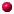Statistical Tables 2001

 Copyright by Dr. Achim Lewandowski (e-mail), Erlangen, beta version 0.3: 30.01.2001

Help:Very important: Your browser must understand JavaScript! Try the latest version of Netscape.If you only see this document and no control buttons, click here.There are two parts: Continuous distribution functions:You can calculate cumulative distribution functions and their inverse functions, generate tables and random numbers. For the discrete random variables it is possible to calculate probability, cumulative probability functions and tables.New: It is possible to generate random numbers. You can use them with Copy and Paste.You must use the decimal point!As input simple numbers but also mathematical more complex expressions are allowed. For example the input of Mu=1 and Sigma=1 for the normal distribution gives the same result as Mu=3*Math.pow(4,1/2)-5 and Sigma=2*Math.sin(Math.PI/2)-1 or Mu=3-2 and Sigma=-1+2*1.If you change the digits or parameters you have to click into the x- (or F(x)-) field before pressing the "x->F(x)"- button.Almost all algorithms were converted from Turbo Pascal to JavaScript (Source: Numerical Recipes in Pascal). The books of Johnson+Kotz (Continuous Distributions I+II, Discrete Distributions) were also used.You are allowed to copy the necessary files (zipped in tables_e.zip (40 k). Unzip the files into one directory and start "stattab.htm". Please do not change anything and always indicate the source.